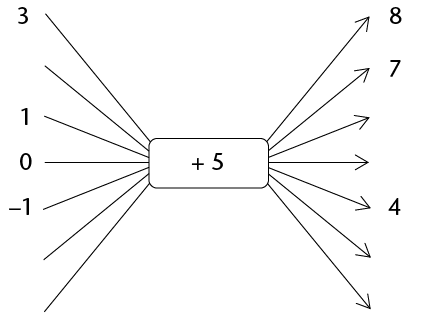Home Practice
For learners and parents For teachers and schools
Textbooks
Full catalogue
Pricing SupportLog in

We think you are located in United States. Is this correct?

# Test yourself now

High marks in science are the key to your success and future plans. Test yourself and learn more on Siyavula Practice.

## 5.6 Chapter summary

• An ordered pair is made of two numbers, and the independent value always comes first. The coordinate pair, $$(x; y)$$, is an example of an ordered pair.
• A table of values lists two groups of numbers:
• The top row lists the independent values (or input values or $$x$$-values).
• The bottom row lists the dependent values (or output values or $$y$$-values).
• The numbers that are above and below each other are related to each other by the equation, and make an ordered pair.
• The numbers that are above and below each other are related to each other by the equation.
• A relationship between two quantities can be shown using a flow diagram, such as the one below. Each input number in a flow diagram has a corresponding output number. The first (top) input number corresponds to the first output number. The second input number corresponds to the second output number, and so on.• A completed flow diagram shows two kinds of information:
• It shows what calculations are done to produce the output numbers.
• It shows which output number is connected to which input number.
• A formula is a mathematical relationship or rule expressed in numbers and letters. A formula is also an equation, which shows how the amounts are related to each other.
• $$x$$ is always the input value for an equation, and $$y$$ is always the output value.
• Each pair of $$x$$- and $$y$$-values that correspond to each other and make the equation true is called a solution of the given equation. A solution to the equation is an ordered pair that fits into the equation. In other words, a solution is a combination of $$x$$- and $$y$$-values that makes one side of the equation equal to the other side.